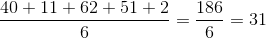## Example Questions

### Example Question #1 : How To Find The Missing Number In A Set

Ryan’s class lined up in order of birthdays. If Ryan is the 11th oldest and 9th youngest, how many students are in his class?

18

20

11

19

21

19

Explanation:

Ryan is the 11th oldest and 9th youngest, so there are 10 students older than him and 8 younger than him. The 10 older plus the 8 younger plus Ryan himself gives a total of 10+8+1 = 19. (The trick is not to double-count Ryan.)

### Example Question #1 : Sets

What is the next integer in this series of numbers?

1, 4, 7, 10, 13, ?

16

14

15

18

17

16

Explanation:

Each number is the previous number + 3.

Example: 1 + 3 = 4

13+3 = 16

### Example Question #3 : How To Find The Missing Number In A Set

If the average and median of the group of integers shown below is equal to x, what is the value of x?

40, 11, 62, x, 20, 51, 2

31

14

26

28

31

Explanation:

First, we must find the average of these 6 numbers. This is done by adding all of them and dividing by the number of values we have (in this case, 6).Next, we have to make sure that this number is also the median.  Since the median is the middle number of our values put in ascending order, we have to put our values in increasing order:

2, 11, 20, 31, 40, 51, 62

When we do this, we see that 31 is indeed the “middle number” so we are happy with choosing 31 as our correct answer

### Example Question #4 : How To Find The Missing Number In A Set

The following series of numbers follows a rule to progress from number to number.  What is the value of Y?

4, 15, 59, Y, 939

329

95

97

789

235

235

Explanation:

Each number from set is:

(Previous Number x 4) – 1

Example: (4 x 4) – 1 = 15

Answer: (59 x 4) – 1 = 235

### Example Question #5 : How To Find The Missing Number In A Set

There is a set of numbers. The mean of this set of numbers is equal to the median.  If four of the numbers are 10, 15, 15, and 17, what is the fifth number?

12

15

18

16

13